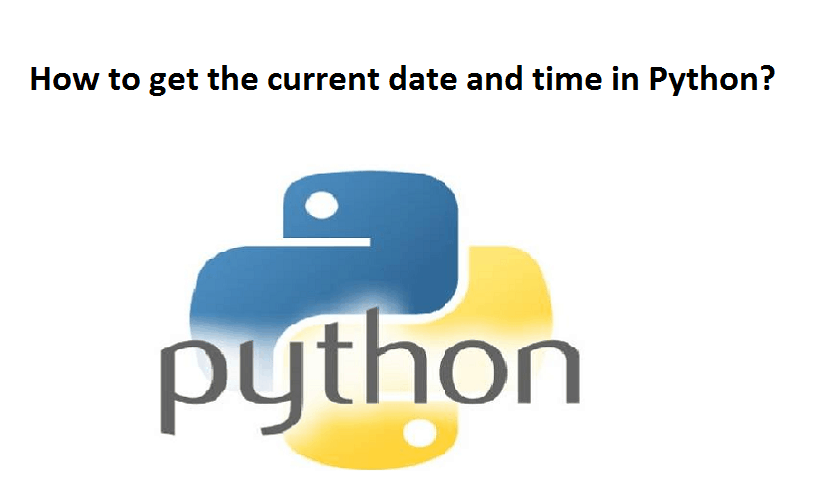# Python Get Current timeIn this tutorial, you will learn to get the current time of your locale as well as various time zones in Python.

There are various ways you can take to get the current time in Python.

## Example 1: Current time using datetime object

```from datetime import datetime

now = datetime.now()

current_time = now.strftime("%H:%M:%S")
print("Current Time =", current_time)```

In the above example, we have imported datetime class from the datetime module. Then, we used the now() method to get a datetime object containing the current date and time.

Using datetime.strftime() method, we then created a string representing the current time.

If you have to make a time object containing the current time, you can do something like this.

```from datetime import datetime

now = datetime.now().time() # time object

print("now =", now)
print("type(now) =", type(now))	```

## Example 2: Current time using the time module

You can also get the current time using the time module.

```import time

t = time.localtime()
current_time = time.strftime("%H:%M:%S", t)
print(current_time)
```

## Example 3: Current time of a timezone

If you need to find the current time of a certain timezone, you can use the pytZ module.

```from datetime import datetime
import pytz

tz_NY = pytz.timezone('America/New_York')
datetime_NY = datetime.now(tz_NY)
print("NY time:", datetime_NY.strftime("%H:%M:%S"))

tz_London = pytz.timezone('Europe/London')
datetime_London = datetime.now(tz_London)
print("London time:", datetime_London.strftime("%H:%M:%S"))```

Please feel free to give your comment if you face any difficulty here.

For more Articles click on the below link.### How to get the current date and time in Python?### Python timestamp to datetime and vice-versa